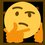# Fundamental Theorem of Calculus Part Two

$\text{Theorem:}\space \boxed{\int _{ a }^{ b }{ f\prime \left( x \right) dx } =f\left( b \right) -f\left( a \right)}\quad \text{(Assuming}\space f\prime \left( x \right) \space \text{is continuous)}$

$\space$

$\text{Riemann Integration: fix}\space n\space \text{and chop}\space \left[ a,b \right] \space \text{into}\space n\space \text{pieces, each piece has length}\space \frac{b-a}{n}=\delta x$

$\text{Notation:}\space x_0=a,\space x_1=a+\delta x,\space x_2=a+2\delta x,\space \cdots,\space x_i=a+i\delta x,\space x_n=a+n\delta x=a+n\left(\frac{b-a}{n}\right)=a+b-a=b$

$\text{On each piece}\space \left[ x_{i-1},x_i \right]\space \text{, choose a point}\space { x }_{ i }^{ \ast }\space \text{, and consider the rectangle of height}\space f\prime\left({ x }_{ i }^{ \ast }\right)\space \text{and of width}\space \delta x$

$\text{Area of rectangle}=\left(\delta x\right)f\prime\left({ x }_{ i }^{ \ast }\right)\quad \text{Take the sum of the areas of all the rectangles}$

$\lim _{ n\rightarrow \infty }{ \sum _{ i=1 }^{ n }{ \left( \delta x \right) f\prime \left( { x }_{ i }^{ \ast } \right) } } \overset { \text{def} }{ = } \int _{ a }^{ b }{ f\prime \left( x \right) dx }$

$\int _{ a }^{ b }{ f\prime \left( x \right) dx }=\lim _{ n\rightarrow \infty }{ \sum _{ i=1 }^{ n }{ \left( \delta x \right) f\prime \left( { x }_{ i }^{ \ast } \right) } }$

$\space$

$\text{By Mean Value Theorem, there is}\space c\space \text{in}\space \left(x_{i-1},x_i \right)\space \text{such that}\space \frac { f\left( { x }_{ i } \right) -f\left( { x }_{ i-1 } \right) }{ { x }_{ i }-{ x }_{ i-1 } } =f\prime \left( c \right)$

$\frac { f\left( { x }_{ i } \right) -f\left( { x }_{ i-1 } \right) }{ \delta x } =f\prime \left( c \right)\quad \text{Let}\space { x }_{ i }^{ \ast }=c\space \text{, then:}$

$\frac { f\left( { x }_{ i } \right) -f\left( { x }_{ i-1 } \right) }{ \delta x } =f\prime \left( { x }_{ i }^{ \ast } \right)$

$\left( \delta x \right) f\prime \left( { x }_{ i }^{ \ast } \right) =f\left( { x }_{ i } \right) -f\left( { x }_{ i-1 } \right)$

$\space$

\begin{aligned} \int _{ a }^{ b }{ f\prime \left( x \right) dx } & = \lim _{ n\rightarrow \infty }{ \sum _{ i=1 }^{ n }{ \left( \delta x \right) f\prime \left( { x }_{ i }^{ \ast } \right) } } \\ \quad & = \lim _{ n\rightarrow \infty }{ \sum _{ i=1 }^{ n }{ \left( f\left( { x }_{ i } \right) -f\left( { x }_{ i-1 } \right) \right) } } \\ \quad & = \lim _{ n\rightarrow \infty }{ \left( f\left( { x }_{ 1 } \right) -f\left( { x }_{ 0 } \right) +f\left( { x }_{ 2 } \right) -f\left( { x }_{ 1 } \right) +\cdots +f\left( { x }_{ n-1 } \right) -f\left( { x }_{ n-2 } \right) +f\left( { x }_{ n } \right) -f\left( { x }_{ n-1 } \right) \right) } \\ \quad & = \lim _{ n\rightarrow \infty }{ \left( f\left( { x }_{ n } \right) -f\left( { x }_{ 0 } \right) \right) } \\ \quad & = \lim _{ n\rightarrow \infty }{ \left( f\left( b \right) -f\left( a \right) \right) } \\ \int _{ a }^{ b }{ f\prime \left( x \right) dx } & = f\left( b \right) -f\left( a \right)\quad ∎ \end{aligned}Note by Gandoff Tan
10 months, 3 weeks ago

This discussion board is a place to discuss our Daily Challenges and the math and science related to those challenges. Explanations are more than just a solution — they should explain the steps and thinking strategies that you used to obtain the solution. Comments should further the discussion of math and science.

When posting on Brilliant:

• Use the emojis to react to an explanation, whether you're congratulating a job well done , or just really confused .
• Ask specific questions about the challenge or the steps in somebody's explanation. Well-posed questions can add a lot to the discussion, but posting "I don't understand!" doesn't help anyone.
• Try to contribute something new to the discussion, whether it is an extension, generalization or other idea related to the challenge.

MarkdownAppears as
*italics* or _italics_ italics
**bold** or __bold__ bold
- bulleted- list
• bulleted
• list
1. numbered2. list
1. numbered
2. list
Note: you must add a full line of space before and after lists for them to show up correctly
paragraph 1paragraph 2

paragraph 1

paragraph 2

[example link](https://brilliant.org)example link
> This is a quote
This is a quote
    # I indented these lines
# 4 spaces, and now they show
# up as a code block.

print "hello world"
# I indented these lines
# 4 spaces, and now they show
# up as a code block.

print "hello world"
MathAppears as
Remember to wrap math in $$ ... $$ or $ ... $ to ensure proper formatting.
2 \times 3 $2 \times 3$
2^{34} $2^{34}$
a_{i-1} $a_{i-1}$
\frac{2}{3} $\frac{2}{3}$
\sqrt{2} $\sqrt{2}$
\sum_{i=1}^3 $\sum_{i=1}^3$
\sin \theta $\sin \theta$
\boxed{123} $\boxed{123}$

Sort by:

In addition I admire your work on the daily problems. Sometimes though it takes some time to wrap my head around it. 😂

- 10 months, 1 week ago

Hey @Gordon Chan, i was wondering how you learned and understand all your knowledge about maths and physics. Can you give me some pointers where to begin? I am in 12. Grade after the summer holidays and chose math and physics because I find it fascinating and challenging. Thanks in advance!

- 10 months, 1 week ago

I find the Internet such a wonderful place in the sense that I can learn many things I want to learn.

- 10 months, 1 week ago

You're totally right! I am lucky to be born in am age where I can access information in a fingersnap. Thank you for reminding me.

- 10 months, 1 week ago